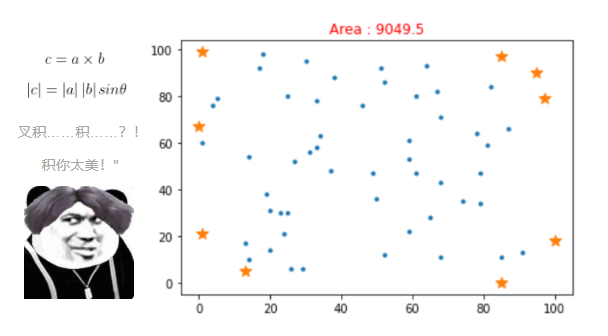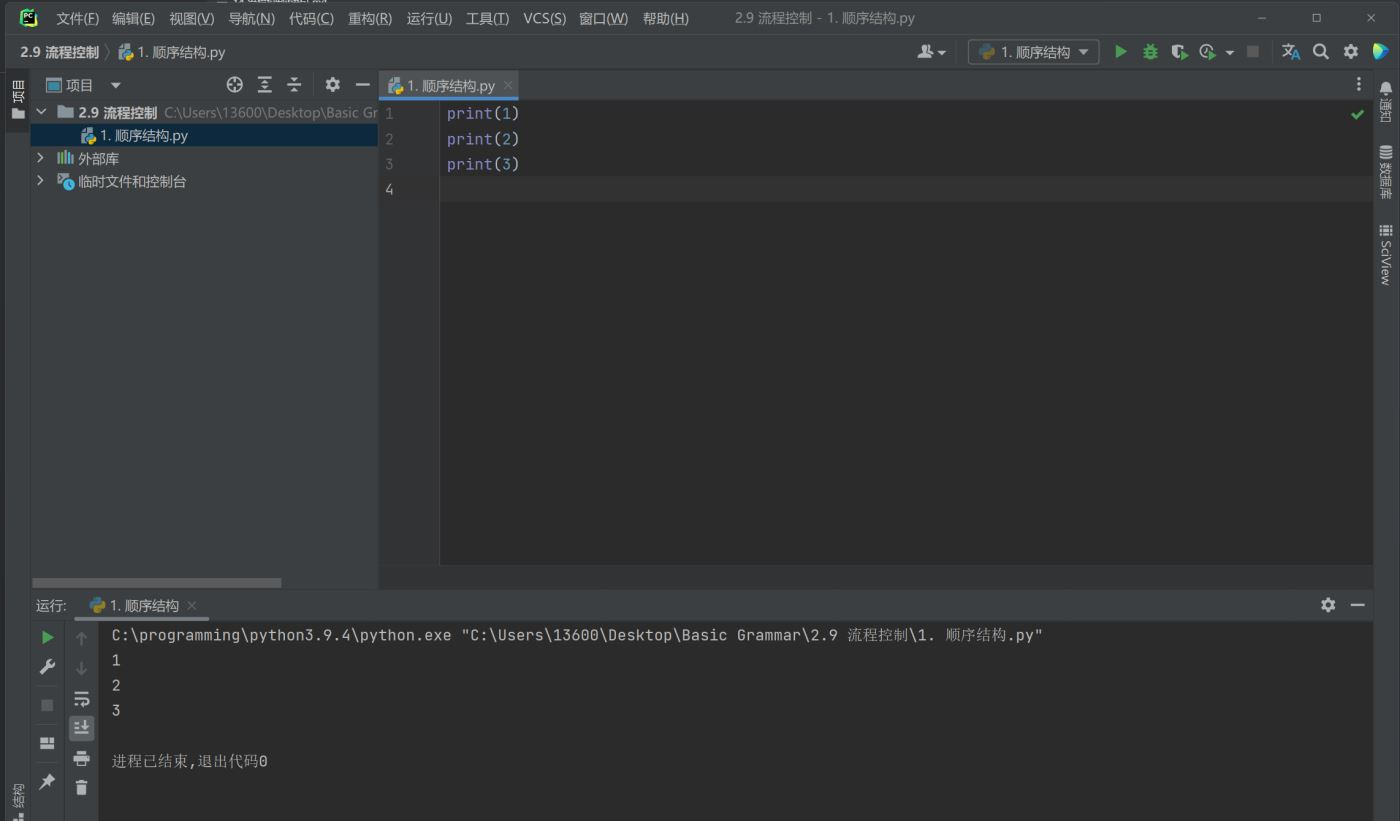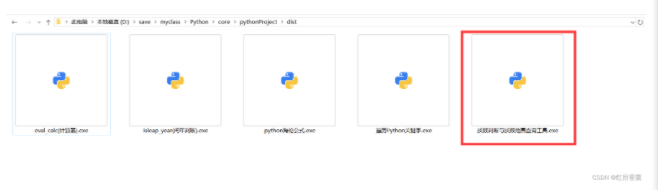# 《从问题到程序：用Python学编程和计算》——2.7　判断和条件控制

+关注继续查看

### 2.7　判断和条件控制

2.7.1　条件判断和逻辑表达式https://yqfile.alicdn.com/c363f0e3f86cee070abadc552943d7b640520f4a.png" >

1）A与B，表示A和B都成立的组合判断；
2）A或B，表示A与B之中至少有一个成立的组合判断；
3）非A，表示A不成立的组合判断。
Python为上面三个逻辑组合关系提供了三个运算符：https://yqfile.alicdn.com/2ff53ef3ebf6b750465db4cdb7f399623e1643da.png" >

Python关于比较运算符和逻辑运算符的优先级和结合性的规定如下：

• 比较运算符的优先级低于算术运算符，因此x + y > x2相当于(x + y) > (x2)，是合法的关系表达式。所有比较运算符的优先级相同，连续的比较表示对相邻运算对象做关系运算之后用and连接，相互为“与”关系。
• 三个逻辑运算符中，一元运算符not的优先级最高，但低于比较运算符，or的优先级最低，and介于两者之间。因此，not x2 > 5 or y < 6 and x y > 8相当于not (x2 > 5) or ((y < 6) and (x y > 8))。

a > 0 and b > 0 and c > 0 and \
a + b > c and b + c > a and a + c > b


2.7.2　if语句（条件语句）

if 表达式:
语句组


if 表达式:
语句组
else:
语句组


# Program to calculate area of triangle
from math import sqrt

a = float(input("Length of edge a: "))
b = float(input("Length of edge b: "))
c = float(input("Length of edge c: "))

if a > 0 and b > 0 and c > 0 and \
a + b > c and b + c > a and a + c > b:
s = (a + b + c) / 2
area = sqrt(s * (s - a) * (s - b) * (s - c))
print("Area of the triangle:", area)
else:
print(a, b, c, "do not form a triangle!")


>>>
Length of edge a: 2
Length of edge b: 5
Length of edge c: 8
2.0 5.0 8.0 do not form a triangle

if语句和程序格式

if语句的扩展形式

if 表达式:
语句组
elif 表达式:
语句组
……
else:
语句组


2.7.3　编程实例

from math import sqrt

print("Program for root(s) of quadratic equetions.")
a = float(input("Coefficient of x**2: "))
b = float(input("Coefficient of x: "))
c = float(input("Constant: "))

d = b**2 - 4 * a * c
if d > 0:
tmp = sqrt(d)
print("Two roots:", (-b + tmp)/2/a,  (-b - tmp)/2/a)
elif d == 0:
print("One root:", -b/2/a)
else:
print("No real root")


# A program calculating total price of some goods

name = input("Please give the name of the good: ")
price = float(input("Price of one piece of the good: "))
amount = int(input("Amount: "))

if price > 0 and amount > 0:
print(amount, "pieces of", name,
"totally: ", price * amount)
else:
print("Error. Price and amount should be positive.")Python判断文件是否存在、访问
Python判断文件是否存在、访问
0 0Python子类判断issubclass和实例判断isinstance
Python子类判断issubclass和实例判断isinstance
0 0【Python】向量叉积和凸包 | 引射线法 | 判断点是否在多边形内部 | 葛立恒扫描法 | Cross Product and Convex Hul

0 0Web 应用程序，python判断文件是否存在,不存在则创建，如何将飞桨中的代码在pycharm中运行
Web 应用程序，python判断文件是否存在,不存在则创建，如何将飞桨中的代码在pycharm中运行
0 0Python定义一个单链表可判断是否为空，计算长度，插入节结点实验
Python定义一个单链表可判断是否为空，计算长度，插入节结点实验
0 0Python基础 if 判断语句详解
Python if 判断语句详解
0 0python:判断字符串类型方法
str对象包括如下用于判断字符串类型的方法: str.isalnum()：是否全为字母或数字 str.isalpha():是否全为字母 str.isdecimal():是否只含十进制数字符号
0 0(Python)判断两个数组是否包含相同元素
(Python)判断两个数组是否包含相同元素
0 0Python数学计算工具2、判断质数、遍历质数
Python数学计算工具2、判断质数、遍历质数
0 0

+ 订阅

【科技少年】Python绘画编程第一课252520

【科技少年】Python基础语法128524422101

Python语言基础 - 函数、面向对象、异常处理5455253

Python语言基础 - 列表、元组、字典、集合5289720

【Python入门】Python数据处理编程基础2736

Python 脚本速查手册